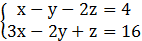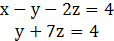### Sample Problem

Solve#### Solution

First use Gaussian elimination to rewrite the system in row-echelon form.E2=E2-3E1Solve for y in terms of z, to obtain

y=4-7z

By back substituting y=4-7z into Equation 1, we can solve for x:

x-(4-7z)-2z=4

x-4+7z-2z=4

x+5z=8

x=8-5z

Finally, let z=a, where a is a real number, and you have the solution

x=8-5a             y=4-7a             z=a

So, every ordered triple in the form of

(8-5a, 4-7a, a) where a is a real number, is a solution of the system.

There are infinite solutions to this system.